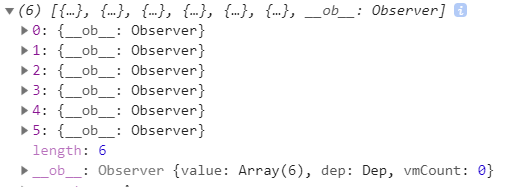# 前言

``````function initState(vm: Component) {
vm._watchers = [];
const opts = vm.\$options;
// ..
if (opts.data) {
initData(vm);
} else {
observe((vm._data = {}), true /* asRootData */);
}
// ..
}``````

``````function initData(vm: Component) {
let data = vm.\$options.data;
data = vm._data = typeof data === "function" ? getData(data, vm) : data || {};
// 校验 options.data 的合法性
// ...
// observe data
observe(data, true /* asRootData */);
}``````

# observe 观察数据

## 代码主体

``````function observe(value: any, asRootData: ?boolean): Observer | void {
if (!isObject(value) || value instanceof VNode) {
return;
}
let ob: Observer | void;
if (hasOwn(value, "__ob__") && value.__ob__ instanceof Observer) {
ob = value.__ob__;
} else if (shouldObserve && !isServerRendering() && (Array.isArray(value) || isPlainObject(value)) && Object.isExtensible(value) && !value._isVue) {
ob = new Observer(value);
}
if (asRootData && ob) {
ob.vmCount++;
}
return ob;
}``````

## 条件判断

``observe(data, true /* asRootData */);``

``````if (!isObject(value) || value instanceof VNode) {
return;
}``````

``hasOwn(value, "__ob__") && value.__ob__ instanceof Observer;``

``````if (hasOwn(value, "__ob__") && value.__ob__ instanceof Observer) {
ob = value.__ob__;
}``````

``shouldObserve && !isServerRendering() && (Array.isArray(value) || isPlainObject(value)) && Object.isExtensible(value) && !value._isVue;``

shouldObserve 默认为 true ，通过调用 toggleObserving 方法来切换其值：

``````function toggleObserving(value: boolean) {
shouldObserve = value;
}``````

shouldObserve 像是一个开关来控制是否来执行 observe 方法，你们在 vue 中找到几处 toggleObserving 开关的使用。

``````function initInjections(vm: Component) {
const result = resolveInject(vm.\$options.inject, vm);
toggleObserving(false);
Object.keys(result).forEach(key => {
defineReactive(vm, key, result[key]);
});
toggleObserving(true);
}``````

isServerRendering 判断是否是 SSR 服务端渲染，本文的运行环境是浏览器中，所以这里判断为 true

``ob = new Observer(value);``

# Observer 观察者对象

## 代码主体

``````class Observer {
constructor(value: any) {
this.value = value;
this.dep = new Dep();
this.vmCount = 0;
def(value, "__ob__", this);
if (Array.isArray(value)) {
// ...
this.observeArray(value);
} else {
this.walk(value);
}
}
walk() {
defineReactive();
}
observeArray() {
observe();
}
}``````

``````this.value = value;
this.dep = new Dep();
this.vmCount = 0;``````

## 对象属性 __ob__

value 对象上新增一个观察属性 __ob__

``def(value, "__ob__", this);``

def 方法内部就是通过定义 数据类型属性

``````Object.defineProperty(obj, key, {
value: val,
enumerable: !!enumerable,
writable: true,
configurable: true
});``````

``this.walk(value);``
``````walk (obj: Object) {
const keys = Object.keys(obj)
for (let i = 0; i < keys.length; i++) {
defineReactive(obj, keys[i])
}
}``````

walk 会遍历 value 上的所有属性（除了 __ob__），并通过 defineReactive 定义 响应式数据

## 数组拦截

``````if (Array.isArray(value)) {
if (hasProto) {
protoAugment(value, arrayMethods);
} else {
copyAugment(value, arrayMethods, arrayKeys);
}
this.observeArray(value);
}``````

``"__proto__" in {};``

``````methodsToPatch.forEach(function(method) {
// cache original method
const original = arrayProto[method];
def(arrayMethods, method, function mutator(...args) {
const result = original.apply(this, args);
const ob = this.__ob__;
let inserted;
switch (method) {
case "push":
case "unshift":
inserted = args;
break;
case "splice":
inserted = args.slice(2);
break;
}
if (inserted) ob.observeArray(inserted);
// notify change
ob.dep.notify();
return result;
});
});``````

``````observeArray (items: Array<any>) {
for (let i = 0, l = items.length; i < l; i++) {
observe(items[i])
}
}``````# defineReactive 响应式数据的定义

``````walk (obj: Object) {
const keys = Object.keys(obj)
for (let i = 0; i < keys.length; i++) {
defineReactive(obj, keys[i])
}
}``````

## 代码主体

``````function defineReactive(obj: Object, key: string, val: any, customSetter?: ?Function, shallow?: boolean) {
const dep = new Dep();

// ...

let childOb = !shallow && observe(val);
Object.defineProperty(obj, key, {
enumerable: true,
configurable: true,
get: function reactiveGetter() {
const value = getter ? getter.call(obj) : val;
// depend ...
return value;
},
set: function reactiveSetter(newVal) {
const value = getter ? getter.call(obj) : val;
// ...
// notify ...
}
});
}``````

## 创建 Dep 对象

``const dep = new Dep();``

## 合法验证

### 对象属性是否可配置

``````const property = Object.getOwnPropertyDescriptor(obj, key);
if (property && property.configurable === false) {
return;
}``````

### 预取值

``````const getter = property && property.get;
const setter = property && property.set;
if ((!getter || setter) && arguments.length === 2) {
val = obj[key];
}``````

``const value = getter ? getter.call(obj) : val;``

## 判断子元素是否需要观察

``let childOb = !shallow && observe(val);``

``````data:{
user:{
name:'eminoda',
child:{
name:'sxh'
}
}
}``````

## 对象属性定义访问器属性

``````Object.defineProperty(obj, key, {
enumerable: true,
configurable: true,
get: function reactiveGetter() {},
set: function reactiveSetter(newVal) {}
});``````

### getter

``const value = getter ? getter.call(obj) : val;``

``````if (Dep.target) {
dep.depend();
if (childOb) {
childOb.dep.depend();
if (Array.isArray(value)) {
dependArray(value);
}
}
}``````

### setter

``````if (newVal === value || (newVal !== newVal && value !== value)) {
return;
}``````

``Number("foo") == Number("foo"); //false``

``````if (process.env.NODE_ENV !== "production" && customSetter) {
customSetter();
}``````

``````if (setter) {
setter.call(obj, newVal);
} else {
val = newVal;
}``````

``````childOb = !shallow && observe(newVal);
dep.notify();``````